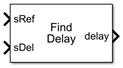# Find Delay

Find delay between two signals

• Library:
• Communications Toolbox / Utility Blocks

•## Description

The Find Delay block finds the delay between a signal and a delayed, and possibly distorted, version of itself. This is useful when you want to compare a transmitted and received signal to find the bit error rate, but do not know the delay in the received signal. This block accepts a column vector or matrix input signal. For a matrix input, the block outputs a row vector, and finds the delay in each channel of the matrix independently. See Delays for more information about signal delays.

## Ports

### Input

expand all

Original reference signal, specified as a vector or matrix. Dimensions and sample times of sRef and sDel must match.

Data Types: `double`

Delayed or distorted version of reference signal, specified as a vector or matrix. Dimensions and sample times of sRef and sDel must match.

Data Types: `double`

### Output

expand all

The output port labeled delay outputs the delay in units of samples.

For a matrix input, the output is a row vector, and finds the delay in each channel of the matrix independently

The delay output is a nonnegative integer less than the Correlation window length.

Data Types: `double`

The chg output port outputs `1` when there is a change from the delay computed at the previous sample, and `0` when there is no change

#### Dependencies

This output port becomes visible only when ```Include "change signal" output port``` is selected.

Data Types: `Boolean`

## Parameters

expand all

The number of samples the block uses to calculate the cross-correlations of the two signals.

As the Correlation window length is increased, the reliability of the computed delay also increases. However, the processing time to compute the delay increases as well.

If you select this option, then the block has an extra output port that emits a value of 1 when the current computed delay differs from the previous computed delay and emits a value of 0 when there is no delay.

Selecting this option causes the block to stop computing the delay after it computes the same delay value for a specified number of samples.

A positive integer specifying how many times the block must compute the same delay before ceasing to update.

#### Dependencies

This field becomes visible only when Disable recurring updates is selected.

## Block Characteristics

 Data Types `double` | `enumerated` | `integera` | `single` Multidimensional Signals `no` Variable-Size Signals `no` a Signed integers only.

expand all

## Tips

• Set Correlation window length sufficiently large so that the computed delay eventually stabilizes at a constant value. When this occurs, the signal from the optional `chg` output port stabilizes at the constant value of zero. If the computed delay is not constant, you should increase Correlation window length. If the increased value of Correlation window length exceeds the duration of the simulation, then you should also increase the duration of the simulation accordingly. If you can roughly estimate the delay, then the Correlation window length will produce a stable delay estimate at four times that value.

• If the cross-correlation between the two signals is broad, then the Correlation window length value should be much larger than the expected delay, or else the algorithm might stabilize at an incorrect value. For example, a CPM signal has a broad autocorrelation, so it has a broad cross-correlation with a delayed version of itself. In this case, the Correlation window length value should be much larger than the expected delay.

• If the block calculates a delay that is greater than 75 percent of the Correlation window length, the signal `sRef` is probably delayed relative to the signal `sDel`. In this case, you should switch the signal lines leading into the two input ports.

• You can make the Find Delay block stop updating the delay after it computes the same delay value for a specified number of samples. To do so, select Disable recurring updates, and enter a positive integer in the Number of constant delay outputs to disable updates field. For example, if you set Number of constant delay outputs to disable updates to `20`, the block will stop recalculating and updating the delay after it calculates the same value `20` times in succession. Disabling recurring updates causes the simulation to run faster after the target number of constant delays occurs.

## Algorithms

The Find Delay block finds the delay by calculating the cross-correlations of the first signal with time-shifted versions of the second signal, and then finding the index at which the cross-correlation is maximized.

## Version History

Introduced before R2006a